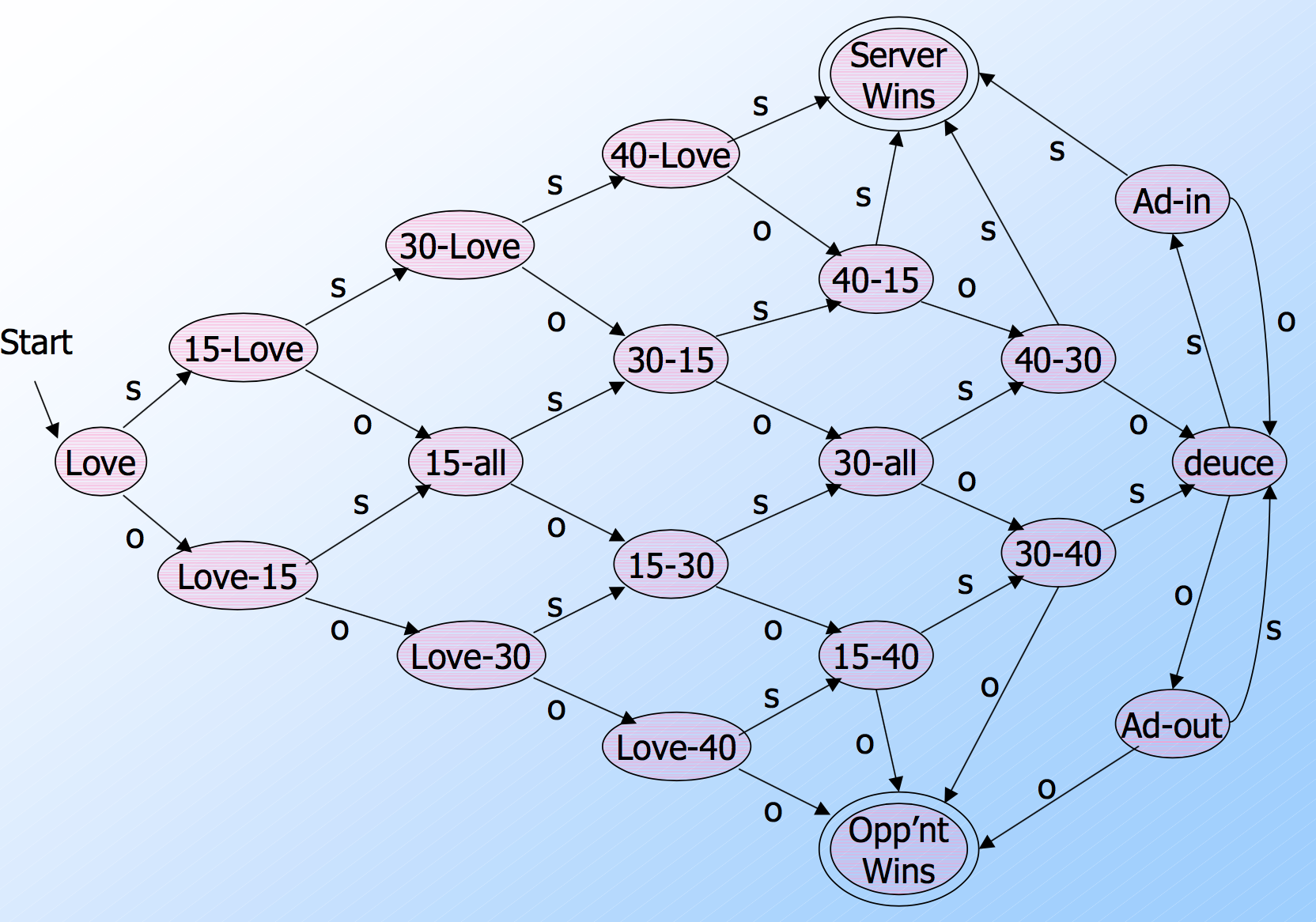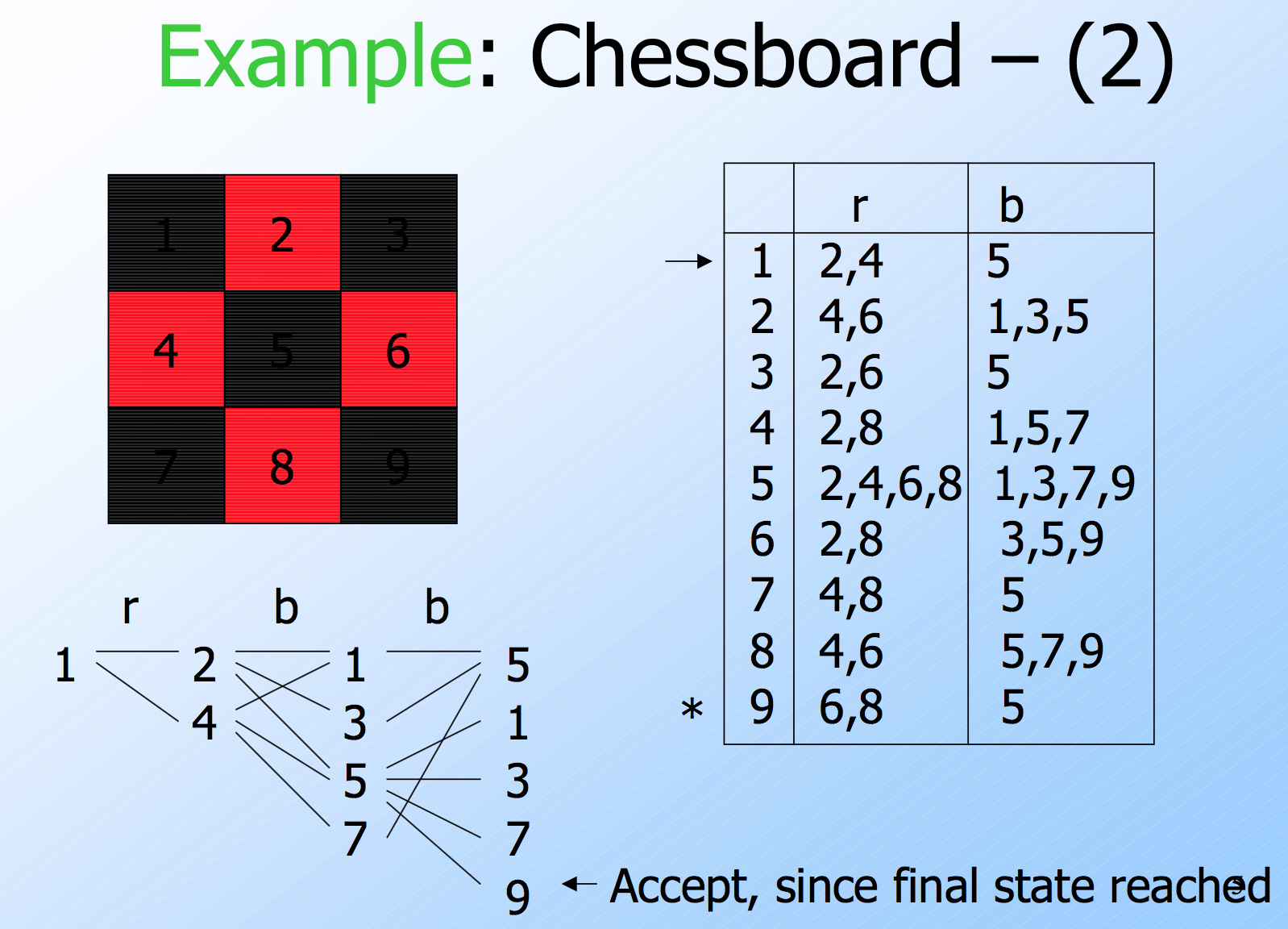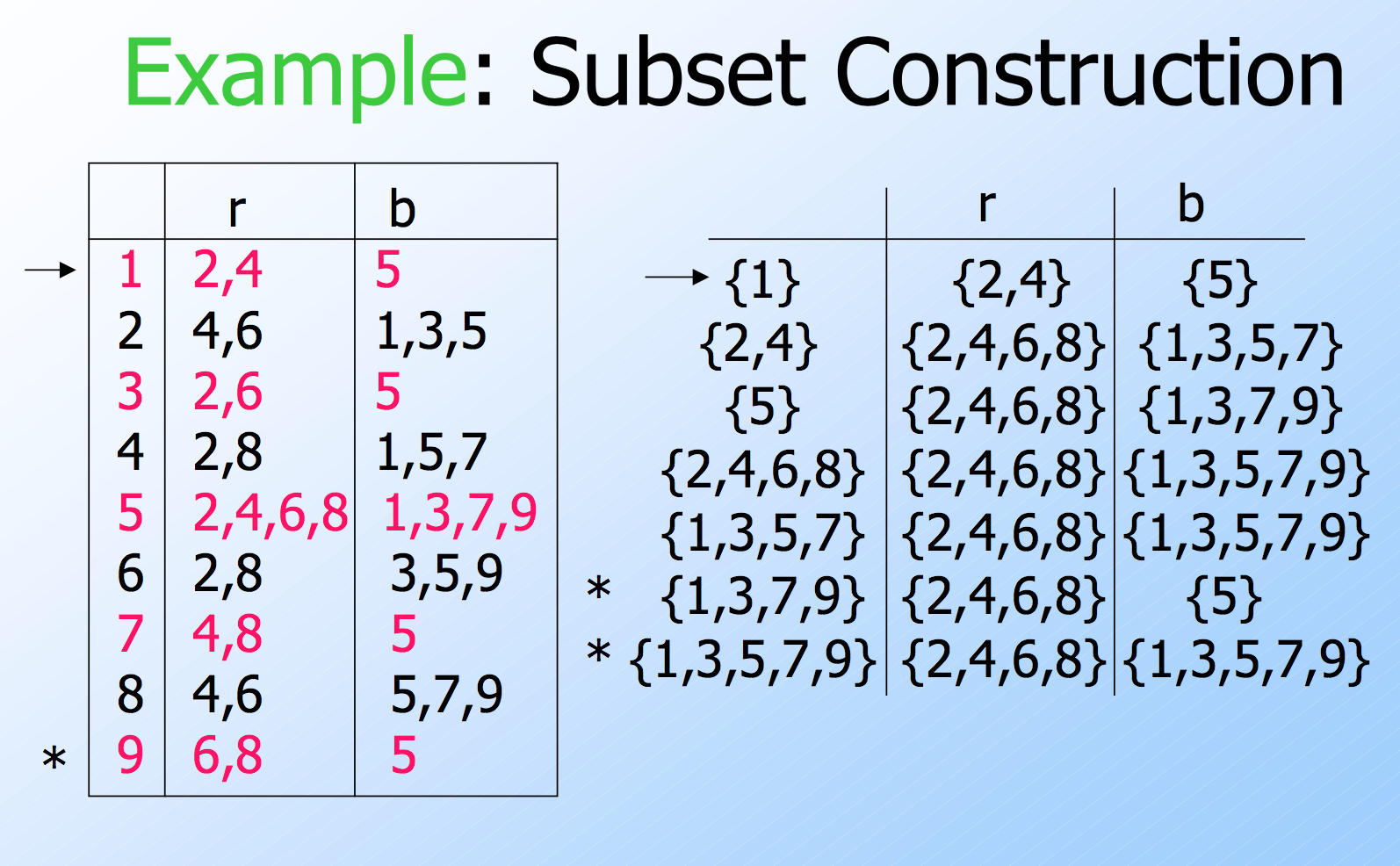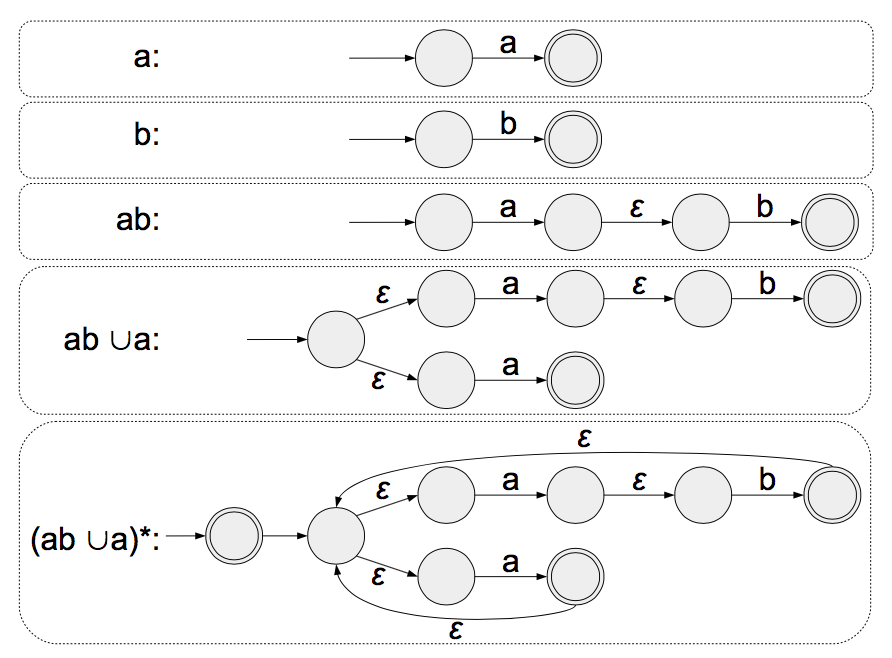Automata itself is a very interesting thesis and Turing Machines which Automata leads to are breathtakingly beautiful.

The Finite Automata part is taught by the Stanford University here but is a little tedious, and the rest part is taught by UC Davis as ECS120 and videos can be found here, the latter UC Davis course is much easier to understand and a lot of fun.

# Introduction

When developing solutions to real problems, we often confront the limitations of what software can do.

• Undecidable things – no program whatever can do it.
• Intractable things – there are programs, but no fast programs.

# Finite Automata

What is a Finite Automaton?

• A formal system.
• Remembers only a finite amount of information.
• Information represented by its state.
• State changes in response to inputs.
• Rules that tell how the state changes in response to inputs are called transitions.Acceptance of Inputs

• Given a sequence of inputs (input string ), start in the start state and follow the transition from each symbol in turn.
• Input is accepted if you wind up in a final (accepting) state after all inputs have been read.

Language of an Automaton

• The set of strings accepted by an automaton A is the language of A.
• Denoted L(A).
• Different sets of final states -> different languages.
• Example: As designed, L(Tennis) = strings that determine the winner.

Nondeterminism

• A nondeterministic finite automaton has the ability to be in several states at once.
• Transitions from a state on an input symbol can be to any set of states.
• Start in one start state.
• Accept if any sequence of choices leads to a final state.
• Intuitively: the NFA always “guesses right.”Equivalence of DFA’s, NFA’s

• A DFA can be turned into an NFA that accepts the same language.
• If δD(q, a) = p, let the NFA have δN(q, a) = {p}.
• Then the NFA is always in a set containing exactly one state – the state the DFA is in after reading the same input.
• Surprisingly, for any NFA there is a DFA that accepts the same language.
• Proof is the subset construction.
• The number of states of the DFA can be exponential in the number of states of the NFA.
• Thus, NFA’s accept exactly the regular languages.

Subset Construction

• Given an NFA with states Q, inputs Σ, transition function $$δ_N$$, state state $$q_0$$, and final states F, construct equivalent DFA with:
• States $$2^Q$$ (Set of subsets of Q).
• Inputs Σ.
• Start state $${q_0}$$.
• Final states = all those with a member of F.
• The transition function δD is defined by: $$δ_D({q_1,...,q_k}, a)$$ is the union over all i = 1,…,k of $$δ_N(q_i, a)$$.# L2: Regular Languages and Non-Deterministic FSMs

A DFA is an NFA, but not every NFA is a DFA.

Theorems:

• Every DFA has an equivalent NFA.
• Every NFA has an equivalent DFA.

Regular Language

A Language that is recognized by some DFA (DFSM) is called a regular language.

Theorems:

• If A and B are regular, then $$A \cup B$$ is regular. Here is proof
• If A and B are regular, then $$A \cap B$$ is regular.
• If A and B are regular, then $$A \circ B$$ is regular. (Concatenation)

# L4: Regular Expression

A regular expression R is:

1. a for some $$a \in \Sigma$$
2. $$\epsilon$$, the empty string
3. $$\Phi$$, the empty set
4. $$(R_1 \cup R_2)$$ where $$R_1$$ and $$R_2$$ are regular expressions
5. $$(R_1 \circ R_2)$$ where $$R_1$$ and $$R_2$$ are regular expressions
6. $$(R_1^*)$$ where $$R_1$$ and $$R_2$$ are regular expressions
• $$R \circ \Phi \equiv \Phi$$ because empty set is equivalent to an NFA which doesn’t accept any string.
• $$\Phi^* \equiv \left\{\epsilon \right\}$$ .
• $$R \cup \Phi \equiv R$$ .
• $$R \circ \epsilon \equiv R$$ .
• $$R \cup \epsilon \equiv R$$ if and only if R contains empty string.

Theorem: A language is regular if and only if it is described by some regular expressions.# L5: Regular Expressions, Regular Languages and Non-Regular Languages

Non-Regular Languages

A string set like this $$\left\{ 0^n1^n : N \ge 0 \right\}$$ can’t be recognized by a DFA, thus not a regular language.

# L6: The Pumping Lemma and Introduction to CFLs

Pumping Lemma:

If A is a regular language, there is a number p (the pumping length) so that if w ∈ A and |w| ≥ p, then w may be divided into three substrings w = xyz, satisfying the conditions:

1. for all i∈N, $$xy^iz∈A$$,
2. |y| > 0, and
3. |xy| ≤ p.